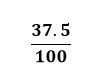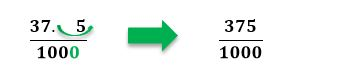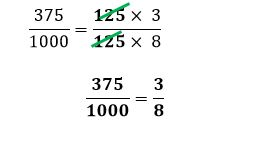# How to convert percentages into fractions

Want to find out how to convert percentages into fractions? We've provided a step-by-step guide of how to convert percentages into fractions to teach you everything you need to know.## Step 1: Change the percentage by dividing it with 100

So, if we want to change 17.25% to a fraction, we express it as:

## Step 2: Change numerator to a whole number

We want to change numerator to a whole number. To do so, move the decimal place to the right until the numerator is a whole number. Add the same number of zeroes on the denominator.

## Step 3: Simplify the fraction

Simplify the fraction by cancelling out common factors.

## Examples of how to convert percentages into fractions

Q1) Serena has completed 37.5% of her project. She plans to graph her progress on a pie chart. What fraction would 37.5% be?

Write 37.5 as the numerator and 100 as the denominator.To make the numerator a whole number, we move one decimal place to the right of 37.5.Simplify the fraction further by cancelling out common factors.This means that Serena has already completed 3/8 of her project.

#### Join today

The fastest way to practice

Unlock our complete testing platform and improve faster that ever.